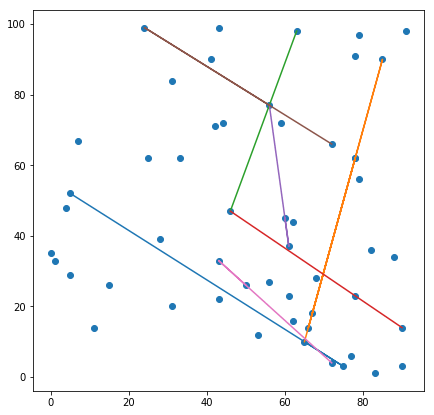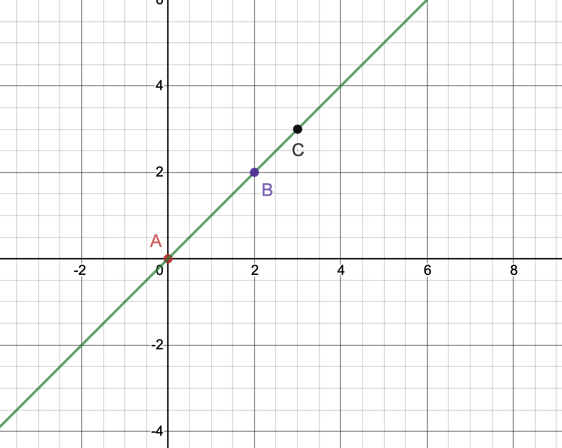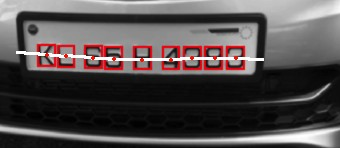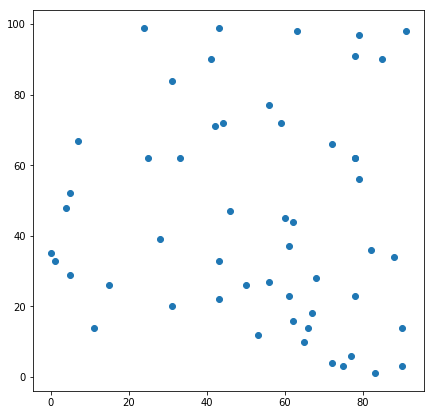# Find clusters of collinear points from a given set of data points

A set of 3 or more points are said to be collinear if they all lie on a straight line as shown in the image below.One common way of checking if three points are collinear is by finding the area of the triangle formed by the points. The area will be zero if the points are collinear.

Area(A,B,C) = 0

Another way is to see if the angle formed by the three points is 180 degrees which is what I used in my number plate segmentation algorithm described in this article. Using the angle to find the collinearity also gives us the advantage of using intervals like:

[175,185] = { angle ∈ ℝ  | 175 <= angle <=185}

The interval can help us find almost collinear points as shown in the image below.But what about finding more than 3 points which are collinear. In this post, I will describe a good way of identifying all the clusters of collinear points in a cartesian plane using the line equation.

## Algorithm

• initialize an array L to hold hash of line equations
• initialize an array CL to holding clusters of lines
• for all distinct points A, B
• compute the line equation y=mx+c where m is slope and c is the intercept
• find the hash of the line equation f(x), check if hash(f(x)) already in L, do not proceed if already present else add it to array L
• initialize an array LINE and add A, B into it.
• for all points C(x3,y3)  ≠ {A, B}
• check if y3-mx3-c = 0, then add C to array LINE as it belongs to the same line
• if the length of LINE > 2, that it contains more than A, B
• add the line into CL

The algorithm complexity is less than O(n3) because of the use of hash function.

## Implementation

The entire notebook implementation can be found here.

Let the points be:

`points = np.array([[42,71],[65,10],[61,23],[46,47],[56,77],[62,44],[78,23],[75,3],[72,4],[43,33],[7,67],[43,22],[88,34],[79,56],[82,36],[56,27],[79,97],[50,26],[1,33],[31,20],[62,16],[15,26],[28,39],[5,52],[78,62],[5,29],[90,3],[61,37],[4,48],[90,14],[59,72],[68,28],[41,90],[63,98],[31,84],[66,14],[78,91],[0,35],[91,98],[43,99],[33,62],[24,99],[77,6],[11,14],[25,62],[53,12],[83,1],[72,66],[44,72],[60,45],[78,62],[85,90],[67,18]])`The implementation of the algorithm described above:

```# get the y=mx+c equation from given points
def get_slope_and_intercept(pointA, pointB):
slope = (pointB - pointA)/(pointB - pointA)
intercept = pointB - slope * pointB
return slope, intercept

# a simple algorithm to find the hash of slope and intercept
def get_unique_id(slope, intercept):
return str(slope)+str(intercept)

lines = []
hash_table = []
for A in points:
for B in points:
if A != B and A != B: #not matching the same points
#find the equation of line
slope, intercept = get_slope_and_intercept(A, B)
line = [A, B]
unique_hash = get_unique_id(slope, intercept)
if unique_hash not in hash_table:
hash_table.append(unique_hash)
for C in points:
if B != C and B != C and A != C and A != C:
# check if this point lies on the same line as A and B
# y - mx - c = 0
rhs = C - slope * C - intercept
if rhs == 0:
line.append(C)
# finally append whatever is found to be in one line, more than 2 points
if len(line) > 2:
lines.append(line)```

The output for our dataset is: